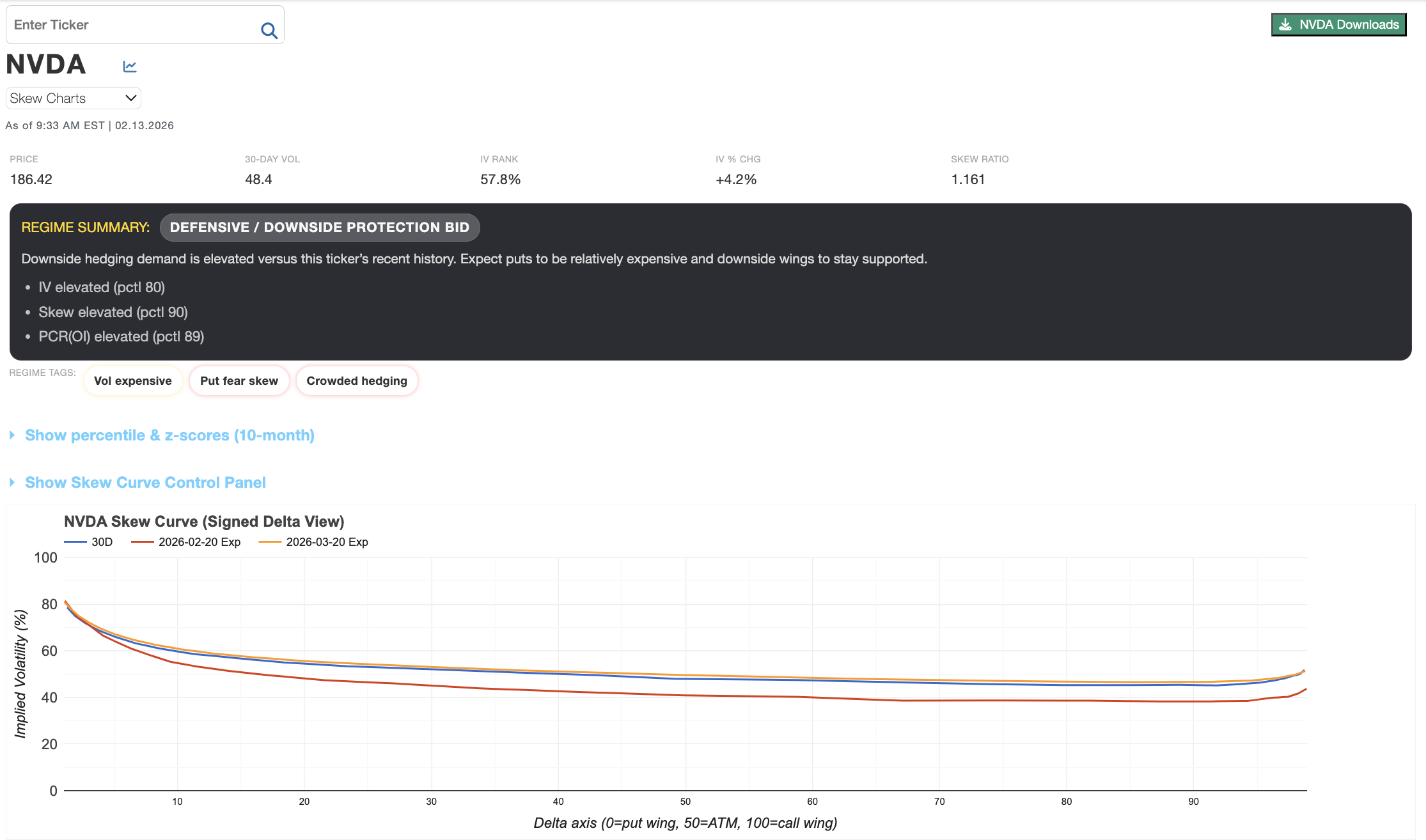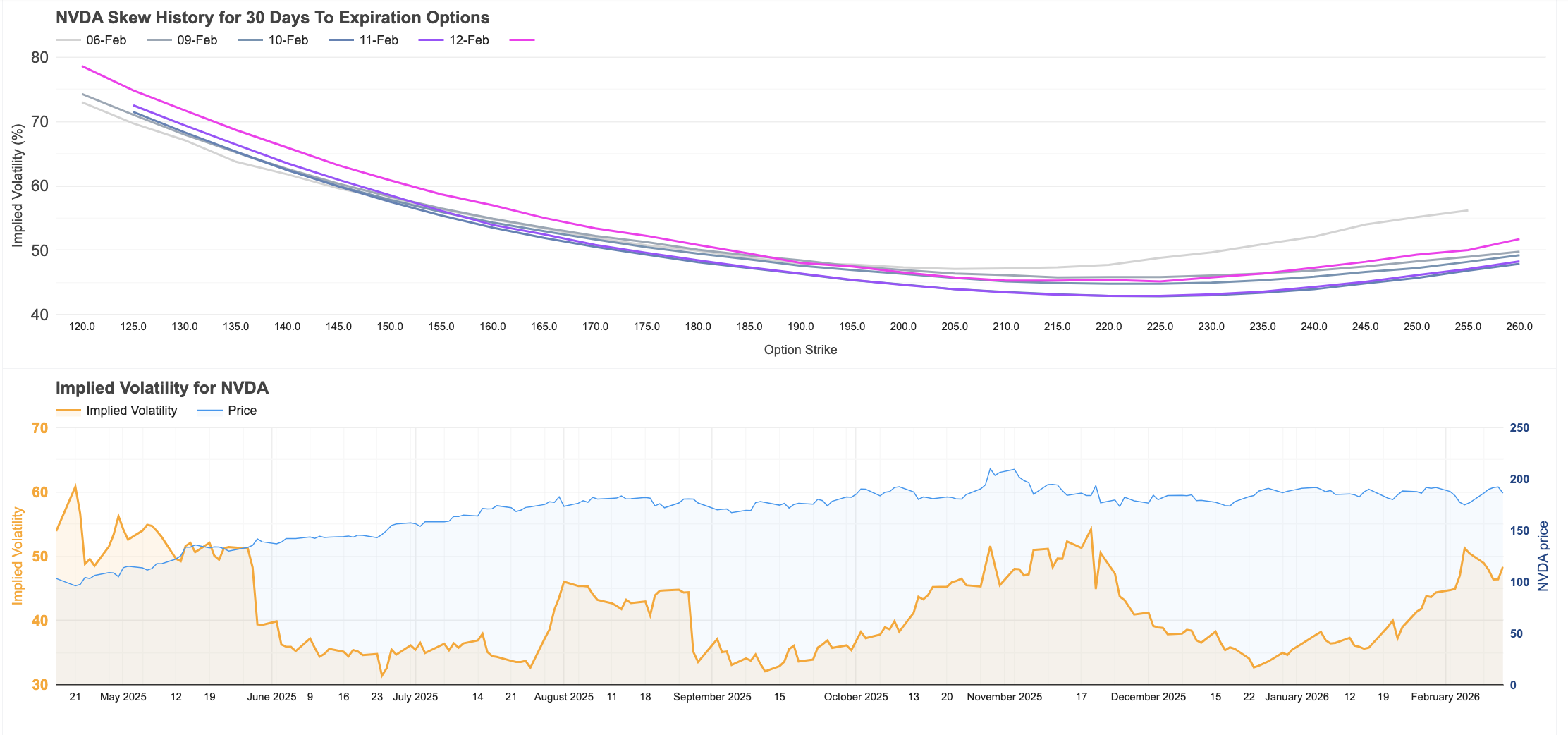Notes on Skew and Delta data:
- Based on the price of options, each stock has an Implied Volatility (IV). The Implied Volatility defines the one standard deviation move over a given period of time.
- "Expected Move" is defined as a One Standard Deviation Move, derived from the stock's current Implied Volatility.
- We take measurements of Put and Call Deltas for options that are one standard deviation out-of-the-money with ~ 30 days to expiration. (The "Delta" for a given contract is defined as the probability that the option will expire in the money.)

Interpretation of Data:
- A stock with a positive Call Skew has option demand skewed toward calls.
- A stock with a negative Call Skew has option demand skewed toward puts.
- The Current Call Skew should be evaluated with respect to its Average Call Skew.
- Sentiment can be evaluated by comparing the Current Call Skew to the Average Call Skew.

The Skew chart displays the Implied Volatility (IV) and Delta for each Out-Of-The-Money put and call contract. Note: The "Delta" at a given contract is the probability that the option will expire in the money.

The Call Skew History chart tracks the "Call Skew", which is the delta of calls at One Standard Deviation above current stock price minus the delta of puts at One Standard Deviation below the current price with 30 days to expiration.

Bollinger Band compression:
- Stocks will generally see their Bollinger Bands (parameters: 10 days & 2 std) compress while price consolidates prior to next breakout.
- Measurement of Bollinger Band width helps people see at a glance what stocks are currently in a compression phase to identify a more ideal time to buy options.
- High IV : BB Width --> Stock is moving less than usual with recent compression in daily price movement.

Implied Volatility Changes:
- Large changes in IV that are not accompanied by large price changes in the underlying are often a prelude to underlying price movements.

Skew Data Table Details:
- Our data looks at all options with less than 94 days to expiration.
- "1 Standard Deviation" is calculated using an average of IVs around the At-The-Money strikes, and then converted to dollars of share price for the given period.